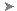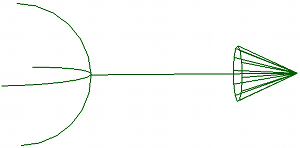# Drag Property Editor

To create a drag control object: Choose GetForceDrag from the Simulate toolbar, GetPrimitiveControl ObjectDrag from the other toolbars, or CreateForceDrag from the Hair toolbar.

To redisplay this property editor: Select the drag object and press Enter.

The Drag forces opposes the motion of ICE particles, rigid bodies, or hair going through a fluid, and depends on the flow of the fluid around the object.

Drag has no effect on Soft body or Cloth simulations.

For more information on forces in general, see Forces [ Simulation and Effects].

To use this force in an ICE tree, see Creating and Applying ICE Forces to ICE Simulations [ICE Guide].Length of the arrow is determined by the Amplitude value.

The basic drag force (Fd) formula is:

Fd = 0.5 * Cd * rf * A * v≤

where:

Cd is the coefficient of drag

rf is the density of the fluid

A is the cross-sectional area of the object

v is the object velocity relative to the fluid velocity

Cd is not a constant but instead depends on the value of a dimensionless constant called the Reynold's number (R). R is a ratio between the inertial property of the fluid that is being pushed out of the way by the object and the viscous drag on the object.

 Name The name of the force. Mute Toggles on/off the force. Amplitude Strength of the drag effect. The Amplitude parameter is a simple strength multiplier, which is the result of 0.5 * Cd * rf. Flow Type You can set the flow type to be either Laminar or Turbulent. A turbulent drag is usually stronger than a Laminar one, but depending on the values you use for the drag force, there may be no discernible difference between these two flow types. Laminar creates a smooth, linear flow in which the fluid is moving along a well-defined and regular path without any turbulence or eddies. Laminar flow is used when R < 1, viscosity dominates and Cd is inversely proportional to R, hence v; therefore, Fd is proportional to v. Turbulent creates a flow in which the fluid moves irregularly and chaotically. Turbulent flow is used when R = 1000~100000, the inertial reaction becomes dominant and Cd is very nearly constant; therefore, Fd is proportional to v 2 . The point at which fluids change their behavior from Laminar to turbulent is the Reynolds number. Area Determines the area used to compute the drag effect on the simulated objects. The basic drag force formula uses the cross-sectional area of the simulated object (Assume Size option) as the scaling factor. If the Assume Size option is on, you can also set the Radius value, which is in Softimage units. This calculates the drag effect using a specific radius around the simulated object. If Assume Size is selected, then DragForce.Area = PI*(Drag.Radius^2) If it's not selected, then DragForce.Area =PI*(Point.Size^2)Except where otherwise noted, this work is licensed under a Creative Commons Attribution-NonCommercial-ShareAlike 3.0 Unported License